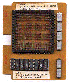Magnetic Core Memory'sSection One - Binary Notation

## Binary Notation

We have said that an artificial brain can deal only with numbers. Of course the human brain can also deal with numbers, but not exclusively with numbers. Human beings, at least in most civilised countries, use for the purposes of recording and calculation what is known as the decimal system of notation, in which any quantity or number can be represented by an appropriate combination of figures or symbols, of which there are ten kinds - the figures 0, 1, 2, 3, 4, 5, 6, 7, 8 and 9.

Most magnetic memories operate on what is known as the 'binary' notation which quantities (numbers) are represented by suitable arrangements of symbols of two kinds. only, namely 0 and 1. Not only quantities, but also letters, words or commands can be represented by means of the binary code. It will be shown later(*) that all numbers between 0 and 9 in the decimal notation can be denoted in the binary code by various combinations of 0 and1 distributed over four magnetic elements. Larger quantities, of course, require a larger number. of elements.

(*) See section - Relation between the Decimal and Binary Codes.